# Using LookUp and LookUpValue

In this tutorial you will learn in what type of data usage situations and how should you use LookUp and in which LookUpValue (and more).

You will learn this by two examples.

Example 1

1.Create a list with exchange rates similar to the one shown below and name it 'EXCHANGE_RATES'.

To do so, you can use 'exchange-rates.xlsx' file (at the bottom of this page).

Pay attention to types and field names.2.Next, create a new app and add a table widget in it

a)

Name the headers as follows: price in PLN, currency, exchange rate, price in currency.

b)

Set A, C and D columns types to 'currency' and set column A as editable.

c)

Next, bind column B with the previously created list. To do so, go to data tab and find your list there and its field 'code'. Drag 'code' field to column B. By doing this you will create a dropdown in the column, from which you will choose the currency.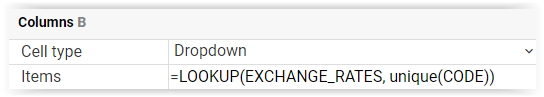Enable the 'allow add rows' option.

d)

In column C we will use LookUpValue formula to get the exchange rate value for the currency chosen in B. First we type in name of the list, then a query - we are looking for values for which the currency code is the same as the one given in B. Lastly we add information about the field from which we want to get the value - in this case it is 'exchange_rate' field.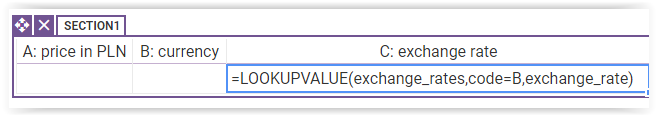e)

Lastly, in column D type '=A/C'. This formula will convert the price in PLN given in A to a correct price in currency.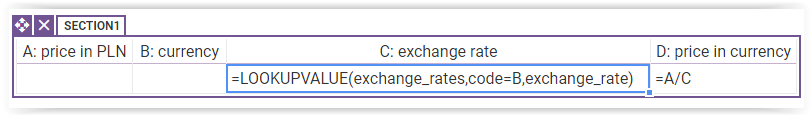3.Check how the app works in preview mode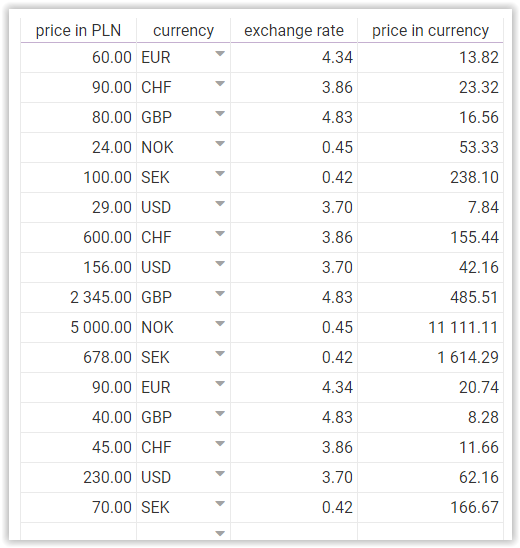App works correctly, in every row after choosing the currency a calculated price in this currency appears.

However it is not a recommended solution, because of the fact that there exist better ones for this situation- faster ones. By repeatedly using LookUpvalue formula - in every row- we have to call the database every time and get data from it.

To use a better method we only need to change a few things in our app.

a)

It should consist of two columns. Bind it with the list by using LookUp formula. You can do it by putting the formula in 'filter' field in table options.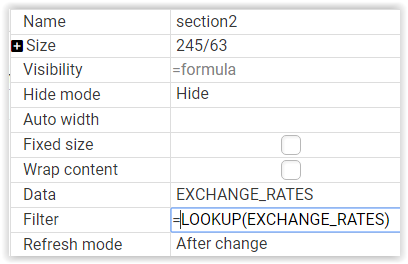Next, both columns bind with the fields from the list (code, exchange_rate).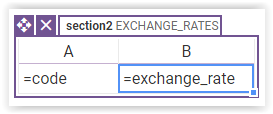b)

Go back to the first table. Delete the previously used formula from column C. We will replace it with the 'Nth' formula. It returns an element from a range/array which is on a given position.

As the range we will put column B from the second table (section2.B). To give the position we will use 'find' formula which returns a position of a given element from a range. In 'find' formula as the range we will give column A values (section2.A) and as the searched value we will put column B value from the first table.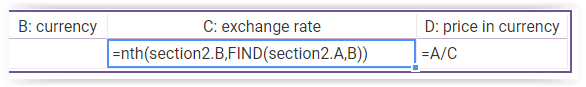As a whole this formula lets us find such a rate exchange(from column B in table 2) for which the currency code( from column A in table 2) is the same as the one chosen previously in column B in table 1.

c)

Let's check how the whole thing works now.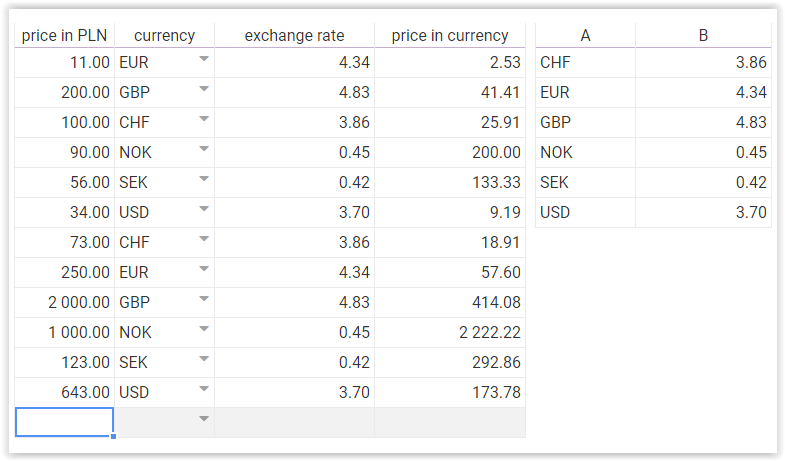Contrary to what we did in the first solution, now we only call the database once - in table 2. Thanks this kind of solution (saving data from the database on the app instance) we save time and make the app work faster.

By analysing this example we now know that, when designing an app which uses little data from the database and many table row it is best to save the data from the database on the app.

Example 2

In order to do this you can use the 'invoice-register.xlsx' file (at the bottom).

It should have a lot of rows because it is an example of an invoice register from a whole year.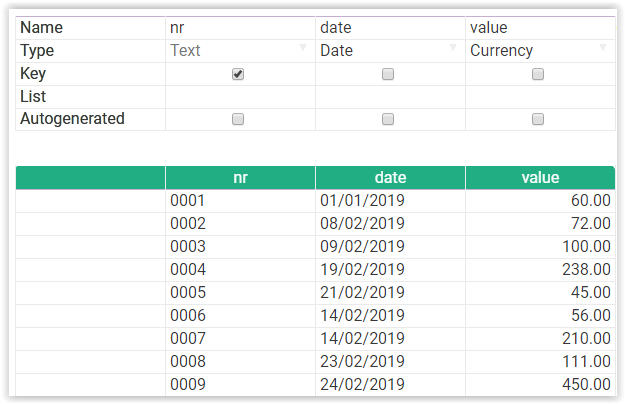2.Create a new app and add a table section

a)

The table should consist of two columns - first one should be a text, editable one and second one not editable with 'currency' type. Name the headers as follows : Invoice nr, is registered. Enable 'allow add rows' option.

b)

Add a second table and bind it with the invoice register list. We will use here the same solution as we used in example 1 - we will get all the data from the database into an additional table.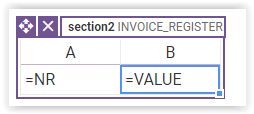Then go back to the first table. We will use a formula corresponding to the one we used in example 1.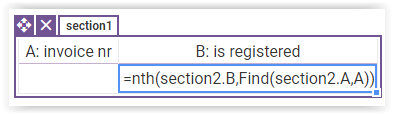Check how the app works in preview mode. You can notice that some items are missing from tabel, which is binded to list. By default you can take 200 items from the list using this method. To remedy it you have to check the 'report' option for this section.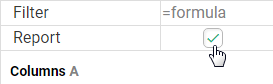3. Formatting (optional step)

a)

In our app we are checking if a chosen invoice has been registered already (if it appears on the created invoice_register list or not). To show which ones hasn't been registered yet we will use conditional formatting. (read here about conditional formatting).

b)

Now, when a given invoice number isn't in the list, formula returns error. The error won't show up, if you add iferror formula before formula in column B in first section, like this:

=iferror(nth(section2.B,find(array(section2.A),A)),0)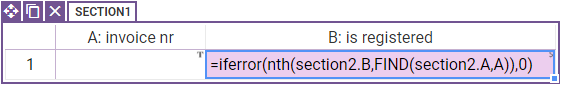Iferror formula checks if given formula returns error. If no, it returns result of formula. If yes, it returns replacement value (in this case 0).

Additionally, check the 'Blank if zero' option. Now, cell will be blank in case of error.

b)

Add a spreadsheet. In it we will create a base for our formatting. Choose one of the spreadsheet's cells and change its color to e.g light red/ pink. Next, choose 'save as' in formatting settings and name the created style.

c)

Go back to table 1. Click on B column and in formatting settings in 'style' field choose 'compute style using formula' option.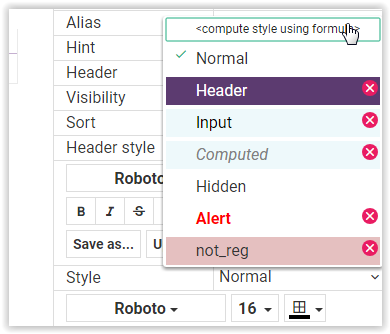In 'style_formula' field put an 'If' formula depending on which cells in column B will change color.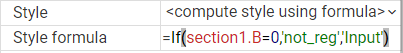If formula returns 0, cell will be in 'not_reg' style.

not_reg is the name of the style created for unregistered invoices.

Input is one of the default styles.

4.Check how the app works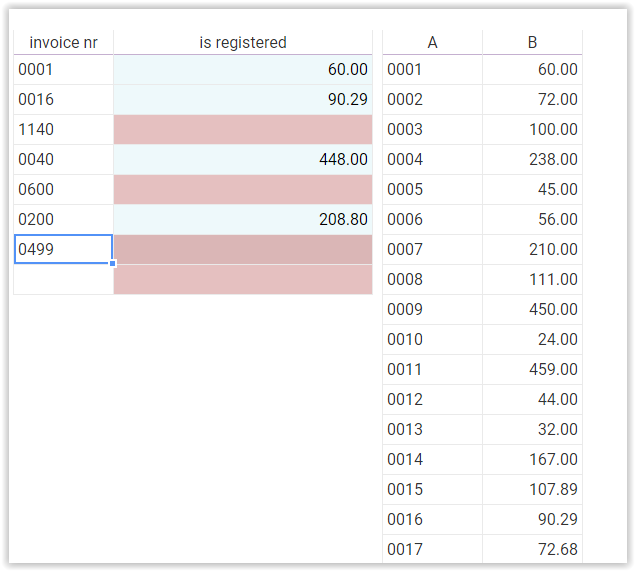As we can see, we don't have too many invoices to check - only a few, because we only want to check if they are registered already. However, we do have many rows in the table- we are getting the whole register.

In a situation in which we have many records in the base, and we only need to find a few of them, we should not get the whole base into a table. Not only all the data is saved on the app then, which makes the app more memory-consuming but it is also slower. In this kind of app structure its best to use a solution shown below.

5.Apply some changes

a)

We will use solution which we rejected in example 1. It means using lookupvalue formula. Hence, we can delete the Nth formula from table 1 and hide or delete table 2.

b)

In column B put a formula resembling the one shown below. By using lookupvalue we will search for such an invoice value that has the same number as the invoice number given in A.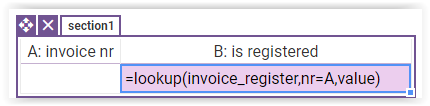Now, if there is no given number in the list, cell will be empty, so need to change 'Style formula' to:

=if(ISEMPTY(section1.B),'not_reg','Input')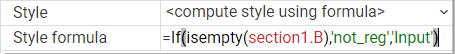Isempty formula returns if a given cell is empty.

c)

Check how this app version works.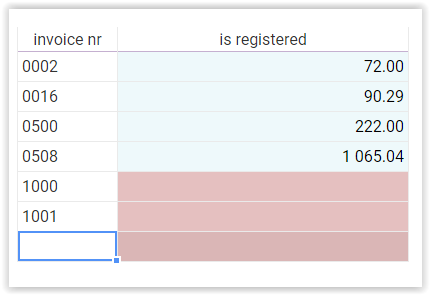Based on this example we can state that searching through a big database every time we add a row is not a bad solution, provided that we don't do this too many times.